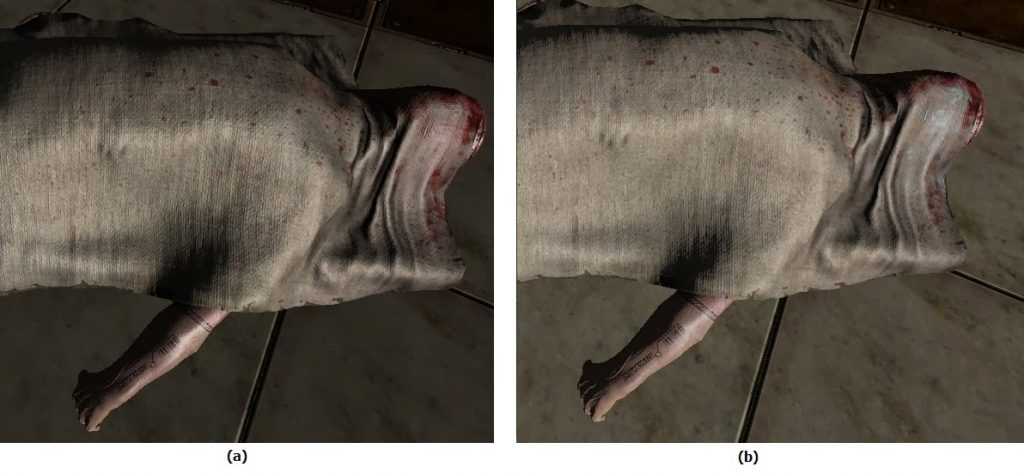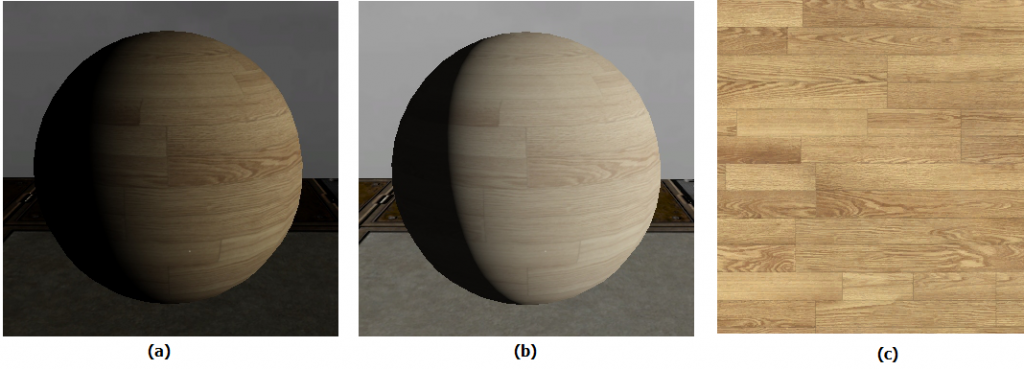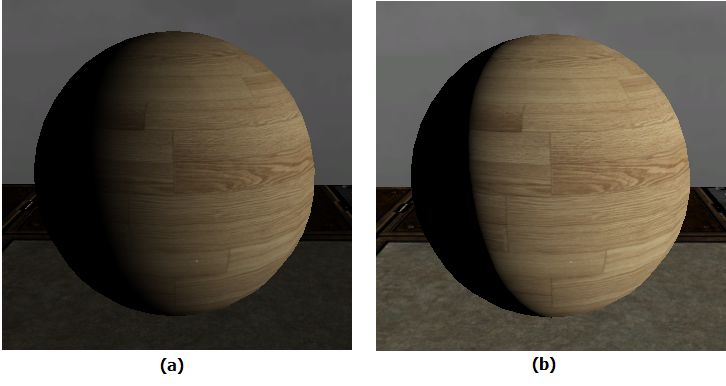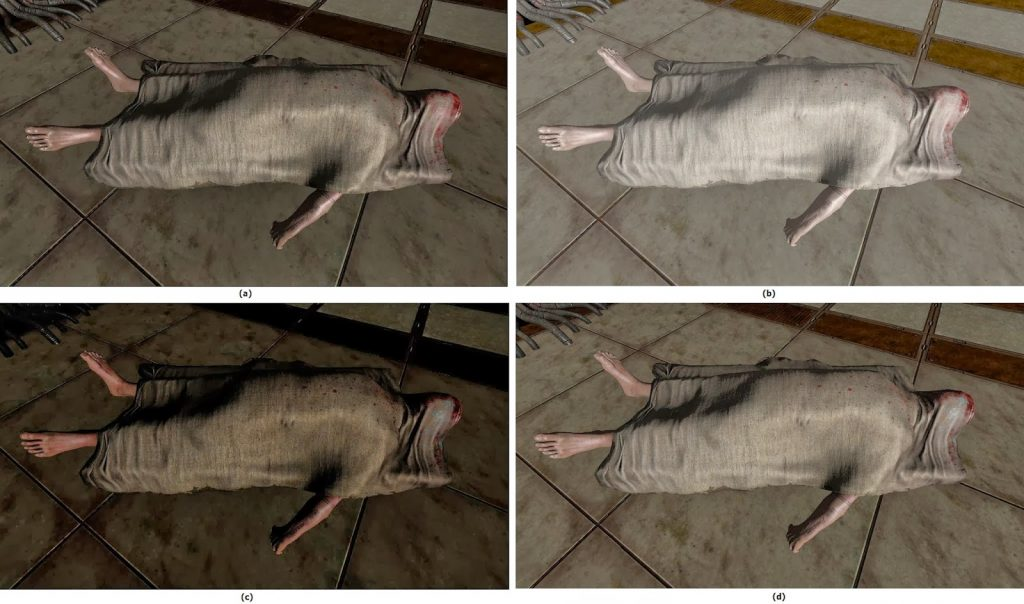# Tech Feature: Linear-space lighting

Originally posted by Peter.

Linear-space lighting is the second big change that has been made to the rendering pipeline for HPL3. Working in a linear lighting space is the most important thing to do if you want correct results.

It is an easy and inexpensive technique for improving the image quality. Working in linear space is not something the makes the lighting look better, it just makes it look correct.(a)  Left image shows the scene rendered without gamma correction (b) Right image is rendered with gamma correction

Notice how the cloth in the image to the right looks more realistic and how much less plastic the specular reflections are.

Doing math in linear space works just as you are used to. Adding two values returns the sum of those values and multiplying a value with a constant returns the value multiplied by the constant.

This seems like how you would think it would work, so why isn’t it?

## Monitors

Monitors do not behave linearly when converting voltage to light. A monitor follows closer to an exponential curve when converting the pixel value. How this curve looks is determined by the monitor’s gamma exponent. The standard gamma for a monitor is 2.2, this means that a pixel with 100 percent intensity emit 100 percent light but a pixel with 50 percent intensity only outputs 21 percent light. To get the pixel to emit 50 percent light the intensity has to be 73 percent.

The goal is to get the monitor to output linearly so that 50 percent intensity equals 50 percent light emitted.

## Gamma correction

Gamma correction is the process of converting one intensity to another intensity which generates the correct amount of light.

The relationship between intensity and light for a monitor can be simplified as an exponential function called gamma decoding.

To cancel out the effect of gamma decoding the value has to be converted using the inverse of this function.

Inversing an exponential function is the inverse of the exponent. The inverse function is called gamma encoding.

Applying the gamma encoding to the intensity makes the pixel emit the correct amount of light.

## Lighting

Here are two images that use simple Lambertian lighting (N * L) .

The left image has a really soft falloff which doesn’t look realistic. When the angle between the normal and light source is 60 degrees the brightness should be 50 percent.  The image on the left is far too dim to match that. Applying a constant brightness to the image would make the highlight too bright and not fix the really dark parts. The correct way to make the monitor display the image correctly is by applying gamma encoding it.(a) Lighting and texturing in gamma space(b) Lighting done in linear space with standard texturing(c) The source texture

Using textures introduces the next big problem with gamma correction. In the left image the color of the texture looks correct but the lighting is too dim. The right image is corrected and the lighting looks correct but the texture, and the whole image, is washed out and desaturated. The goal is to keep the colors from the texture and combining it with the correct looking lighting.

## Pre-encoded images

Pictures taken with a camera or paintings made in Photoshop are all stored in a gamma encoded format. Since the image is stored as encoded the monitor can display it directly. The gamma decoding of the monitor cancels out the encoding of the image and linear brightness gets displayed. This saves the step of having to encode the image in real time before displaying it.

The second reason for encoding images is based on how humans perceive light. Human vision is more sensitive to differences in shaded areas than in bright areas. Applying gamma encoding expands the dark areas and compresses the highlights which results in more bits being used for darkness than brightness. A normal photo would require 12 bits to be saved in linear space compared to the 8 bits used when stored in gamma space. Images are encoded with the sRGB format which uses a gamma of 2.2.

Images are stored in gamma space but lighting works in linear space, so the image needs to be converted to linear space when they are loaded into the shader. If they are not converted correctly there will be artifacts from mixing the two different lighting spaces. The conversion to linear space is done by applying the gamma decoding function to the texture.(a) All calculations have been made in gamma space  (b) Correct texture and lighting, texture decoded to linear space and then all calculations are done before encoding to gamma space again

## Mixing light spaces

Gamma correction a term is used to describe two different operations, gamma encoding and decoding. When learning about gamma correction it can be confusing because word is used to describe both operations.

Correct results are only achieved if both the texture input is decoded and then the final color is encoded. If only one of the operations is used the displayed image will look worse than if none of them are.(a) No gamma correction, the lighting looks incorrect but the texture looks correct. (b) Gamma encoding of the output only, the lighting looks correct but the textures becomes washed out(c)  Gamma decoding only, the texture is much darker and the lighting is incorrect. (d) Gamma decoding of texture and gamma encoding of the output, the lighting and the texture looks correct.

## Implementation

Implementing gamma correction is easy. Converting an image to linear space is done by appling the gamma decoding function. The alpha channel should not be decoded, as it is already stored in linear space.

// Correct but expensive way

vec3 linear_color = pow(texture(encoded_diffuse,  uv).rgb, 2.2);

// Cheap way by using power of 2 instead

vec3 encoded_color = texture(encoded_diffuse,  uv).rgb;

vec3 linear_color = encoded_color * encoded_color;

Any hardware with DirectX 10 or OpenGL 3.0 support can use the sRGB texture format. This format allows the hardware to perform the decoding automatically and return the data as linear. The automatic sRGB correction is free and give the benefit of doing the conversion before texture filtering.

To use the sRGB format in OpenGL just pass GL_SRGB_EXT instead of GL_RGB to glTexImage2D as the format.

After doing all calculations and post-processing the final color should then to be correct by applying gamma encoding with a gamma that matches the gamma of the monitor.

vec3 encoded_output = pow(final_linear_color, 1.0 / monitor_gamma);

For most monitors a gamma of 2.2 would work fine. To get the best result the game should let the player select gamma from a calibration chart.

This value is not the same gamma value that is used to decode the textures. All textures are be stored at a gamma of 2.2 but that is not true for monitors, they usually have a gamma ranging from 2.0 to 2.5.

## When not to use gamma decoding

Not every type of texture is stored as gamma encoded. Only the texture types that are encoded should get decoded. A rule of thumb is that if the texture represents some kind of color it is encoded and if the texture represents something mathematical it is not encoded.

• Diffuse, specular and ambient occlusion textures all represent color modulation and need to be decoded on load
• Normal, displacement and alpha maps aren’t storing a color so the data they store is already linear

## Summary

Working in linear space and making sure the monitor outputs light linearly is needed to get properly rendered images. It can be complicated to understand why this is needed but the fix is very simple.

• When loading a gamma encoded image apply gamma decoding by raising the color to the power of 2.2, this converts the image to linear space
• After all calculations and post processing is done (the very last step) apply gamma encoding to the color by raising it to the inverse of the gamma of the monitor

If both of these steps are followed the result will look correct.

## References

https://en.wikipedia.org/wiki/Gamma_correction
http://http.developer.nvidia.com/GPUGems3/gpugems3_ch24.html
http://filmicgames.com/archives/category/gamma